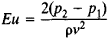# Euler Number

Also found in: Wikipedia.
The following article is from The Great Soviet Encyclopedia (1979). It might be outdated or ideologically biased.

## Euler Number

a similarity criterion used in the study of the motion of liquids or gases.

The Euler number Eu characterizes the relation between the pressure forces acting on an elementary volume of a liquid or gas and the inertia forces. It is defined by the formula(sometimes 2pv2), where p2 and p1 are the pressures at two characteristic points of the flow (or of a body moving in it), ρv2/2 is the velocity head, ρ is the density of the liquid or gas, and v is the fluid velocity (or the velocity of the body).

In the case of liquid flows with cavitation, the analogous criterion is called the cavitation number κ = 2(p0ps)/ρv2, where p0 is the characteristic pressure and ps is the saturation vapor pressure of the liquid.

For compressible gas flows the relation between the Euler number in the form Eu = 2pv2 and two other similarity criteria—the Mach number M and the ratio of the specific heats of the medium γ—is given by the equation Eu = 2/γM2, where γ = cp/cv (cp is the specific heat at constant pressure, and cv is the specific heat at constant volume).

It should be noted that in the American literature two Euler numbers are often used. Euler number 1 equals the friction head divided by two times the velocity head, and Euler number 2 is equal to two times the Fanning friction factor.

The Euler number is named for L. Euler.

References in periodicals archive ?
The features included Perimeter, Area, Major axis length, Minor axis length, Filled area, Orientation, Equiv diameter, Euler number, Solidity, Extent, Eccentricity and Convex Area (Russ, 1999).
It is well-known that numbers of up-down permutations are Euler numbers. Suppose E(n) is the nth Euler number, then |[C[C.sub.=2[n-1]] | = E(2n).
In fact, the computation of the Euler number in virtual planar sections can be replaced by counting connected components or tangent points, as suggested in Schladitz etal.
The logarithmic mean is linked with the Euler number by a simple and elegant formula
 Hirzebruch, F., and Hofer, T., 1990, On the Euler number of an orbifold, Math.
Descartes discovered that there is a connection between the total defect, T, and the Euler number V - E + F, where V, E, F denote the number of vertices, edges and faces of the given polyhedron.
Manually determined flower counts from Plots A and B counted for every date were regressed with values determined by the automated method of flower percentage and Euler number using the linear regression function in Quattro Pro for Windows 3.1x version 7 (Corel Corp.
These are: volume V, surface area S, integral of mean curvature M and Euler number [chi].
where [B.sub.k] := [B.sub.k](0) is the k-th Bernoulli number and [E.sub.k] := [E.sub.k](1) is the k-th Euler number. The Bernoulli numbers may also be calculated from
In 3D, they are, up to constants, volume fraction [V.sub.v], surface area density [S.sub.v], density of the integral of mean curvature [M.sub.v], and Euler number density [[chi.sub.v].
On the one hand, they include the widely used volume V, surface area S, and Euler number [chi].
An important insight is that, in 3D, complementarity according to a consistency relation for the Euler number differs from the complementarity as conventionally defined by the discrete Jordan-Brouwer theorem.

Site: Follow: Share:
Open / Close